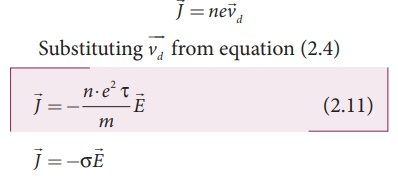Home | | Physics 12th Std | Microscopic model of current

# Microscopic model of current

Physics : Current Electricity : Microscopic model of current

Microscopic model of current

Consider a conductor with area of cross section A and an electric fieldapplied from right to left. Suppose there are n electrons per unit volume in the conductor and assume that all the electrons move with the same drift velocityd as shown in Figure 2.5.The drift velocity of the electrons = vd The electrons move through a distance dx within a small interval of dtSince A is the area of cross section of the conductor, the electrons available in the volume of length dx is = volume ├Ś number per unit volumeSubstituting for dx from equation (2.7) in (2.8)= (A vd dt ) n

Total charge in volume element dQ = (charge) ├Ś (number of electrons in the volume element)## Current density (J)

The current density ( J ) is defined as the current per unit area of cross section of the conductor.

J = I/AThe above expression is valid only when the direction of the current is perpendicular to the area A. In general, the current density is a vector quantity and it is given byBut conventionally, we take the direction of (conventional) current density as the direction of electric field. So the above equation becomeswhere Žā = ne2Žä /m is called conductivity. The equation 2.12 is called microscopic form of ohmŌĆÖs law.

The inverse of conductivity is called resistivity (Žü) [Refer section 2.2.1].### EXAMPLE 2.3

A copper wire of cross-sectional area 0.5 mm2 carries a current of 0.2 A. If the free electron density of copper is 8.4 ├Ś 1028 m-3 then compute the drift velocity of free electrons.

### Solution

The relation between drift velocity of electrons and current in a wire of cross-sectional area A is

vd = I/ ne Avd = 0.03 x 10-3 m s-1

### EXAMPLE 2.4

Determine the number of electrons flowing per second through a conductor, when a current of 32 A flows through it.

### Solution

I = 32 A , t = 1 s

Charge of an electron, e = 1.6 ├Ś 10-19 C

The number of electrons flowing per second, n =?

I = q/t = ne/t

n = It/e

n = 32├Ś1 / 1 .6├Ś10ŌłÆ19 C

n = 20 ├Ś 1019 = 2 ├Ś 1020 electrons

Study Material, Lecturing Notes, Assignment, Reference, Wiki description explanation, brief detail
12th Physics : Current Electricity : Microscopic model of current |